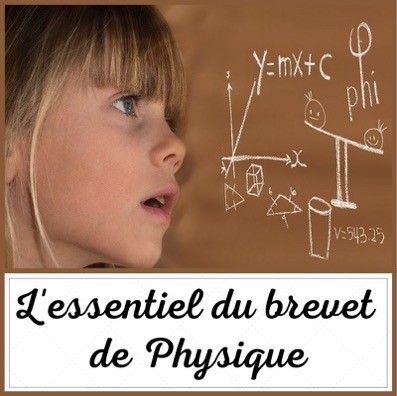# PHYSICAL PATENT: THE ESSENTIAL

II. SPEED - KINETIC ENERGY

II.1. The speed of an object:

La speed is a quantity which measures the ratio of an evolution to time.

where v (speed) is in m / s or ms-1 or km / h or km.h-1 ; d (the distance traveled) in m or km and t (the duration of the trip) is in s or h. To convert km / h to m / s, divide by 3,6 or 1m / s = 3,6 km / h

By extension :

Examples : a marathon runner covers the first 30 kilometers in 1 hour. Its speed is 30 km / h or 30 km. h-1

II.2. The kinetic energy of an object:

THEkinetic energy of an object in translational motion is the energy that a body possesses due to its movement in relation to a given frame of reference. Its value therefore depends on the choice of this repository.

where Ec is in Joule; m in kg or g; v in m / s or km / h

Examples : A 5000 kg bus rolls at 8 m. s-1 . The value of the kinetic energy of this coach is 1/2 times 5 times 0002 = 160 J

Quote on physics:

“Poetry is the mathematics of language and existence, the novel is its physics.”

Marc Gendron

Continuation of the article, electricity, page 3